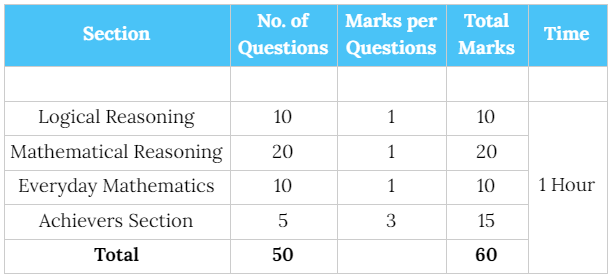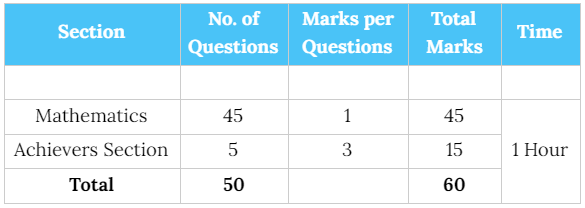The International Mathematics Olympiad (IMO) is a Mathematics competition for students of class 1 to class 12. It is conducted by Science Olympiad Foundation (SOF) with the aim of identifying and nurturing future scientists, technologists and IT talent at school level.

### Exam Pattern

Level-1 ExamLevel-2 ExamStudents fulfilling either of the following 3 criteria's will qualify for 2nd level Olympiads exams:

• Top 5% of students, class wise who appear for the 1st level exam. Due priority to marks scored in different sections will be given to determine ranks in case of a tie of marks.
• From each Zone - top 25 rank holders from each class.
• Class topper from each participating school where at least 10 students from a class appear in the exam & scores 50% qualifying marks.

### Exam Syllabus - Class 5

Logical Reasoning: Patterns, Analogy and Classification, Geometrical Shapes, Mirror and Water Images, Direction Sense Test, Ranking Test, Alphabet Test, Logical Sequence of Words, Puzzle Test, Coding-Decoding. Mathematical Reasoning: Numerals, Number names and Number Sense (7 and 8 digit numbers), Computation Operations, Fractions and Decimals, Measurement of Length, Weight, Capacity, Time, Temperature and Money, Conversions, Geometrical Shapes and Solids, Angles, Perimeter of Various Shapes & Area of Rectangle and Square, Symmetry, Data Handling. Everyday Mathematics: The syllabus of this section will be based on the Syllabus of Mathematical Reasoning. Achievers Section: Higher Order Thinking Questions - Syllabus as per Mathematical Reasoning.

Coming Soon.

### Exam Syllabus - Class 8

Logical Reasoning: Verbal and Non-Verbal Reasoning. Mathematical Reasoning: Rational Numbers, Squares and Square Roots, Cubes and Cube Roots, Exponents and Powers, Comparing Quantities, Algebraic Expressions and Identities, Linear Equations in One Variable, Understanding Quadrilaterals, Constructions, Mensuration, Visualising Solid Shapes, Data Handling, Direct and Inverse Variations, Factorisation, Introduction to Graphs, Playing with Numbers. Everyday Mathematics: The syllabus of this section will be based on the Syllabus of Mathematical Reasoning. Achievers Section: Higher Order Thinking Questions - Syllabus as per Mathematical Reasoning.